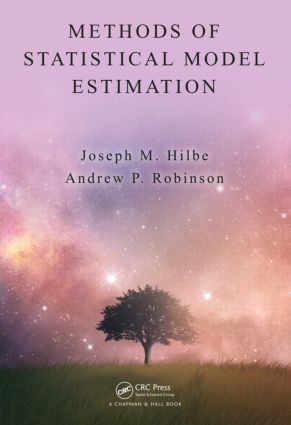# Methods of Statistical Model Estimation

## 1st Edition

Chapman and Hall/CRC

255 pages | 13 B/W Illus.

##### Purchasing Options:\$ = USD
Hardback: 9781439858028
pub: 2013-05-28
\$105.00
x
eBook (VitalSource) : 9780429107221
pub: 2016-04-19
from \$49.48

FREE Standard Shipping!

### Description

Methods of Statistical Model Estimation examines the most important and popular methods used to estimate parameters for statistical models and provide informative model summary statistics. Designed for R users, the book is also ideal for anyone wanting to better understand the algorithms used for statistical model fitting.

The text presents algorithms for the estimation of a variety of regression procedures using maximum likelihood estimation, iteratively reweighted least squares regression, the EM algorithm, and MCMC sampling. Fully developed, working R code is constructed for each method. The book starts with OLS regression and generalized linear models, building to two-parameter maximum likelihood models for both pooled and panel models. It then covers a random effects model estimated using the EM algorithm and concludes with a Bayesian Poisson model using Metropolis-Hastings sampling.

The book's coverage is innovative in several ways. First, the authors use executable computer code to present and connect the theoretical content. Therefore, code is written for clarity of exposition rather than stability or speed of execution. Second, the book focuses on the performance of statistical estimation and downplays algebraic niceties. In both senses, this book is written for people who wish to fit statistical models and understand them.

See Professor Hilbe discuss the book.

### Reviews

"This book is a concise volume of statistical methods associated with parametric models. With a rich set of R codes, the book contains full demonstration of how to apply the parametric statistical models to obtain desired results of analyses with minimal theoretical details. … a useful reference book for a graduate course on statistical models using a standard textbook … many illustrative samples are truly easy to understand. This book is also handy for understanding the algorithm used in statistical model fitting, using the R programming language."

—Jae-kwang Kim, Biometrics, March 2014

### Table of Contents

Programming and R

Introduction

R Specifics

Programming

Making R Packages

Further Reading

Statistics and Likelihood-Based Estimation

Introduction

Statistical Models

Maximum Likelihood Estimation

Interval Estimates

Simulation for Fun and Profit

Ordinary Regression

Introduction

Least-Squares Regression

Maximum-Likelihood Regression

Infrastructure

Conclusion

Generalized Linear Models

Introduction

GLM: Families and Terms

The Exponential Family

The IRLS Fitting Algorithm

Bernoulli or Binary Logistic Regression

Grouped Binomial Models

Constructing a GLM Function

GLM Negative Binomial Model

Offsets

Dispersion, Over and Under

Goodness-of-Fit and Residual Analysis

Weights

Conclusion

Maximum Likelihood Estimation

Introduction

MLE for GLM

Two-Parameter MLE

Panel Data

What Is a Panel Model?

Fixed-Effects Model

Random-Intercept Model

Handling More Advanced Models

The EM Algorithm

Further Reading

Model Estimation Using Simulation

Simulation: Why and When?

Synthetic Statistical Models

Bayesian Parameter Estimation

Discussion

Bibliography

Index

Exercises appear at the end of each chapter.

### About the Authors

#### Joseph Hilbe

Florence, AZ, USA

Learn more about Joseph Hilbe >>

Joseph M. Hilbe is a Solar System Ambassador with NASA's Jet Propulsion Laboratory at the California Institute of Technology, an adjunct professor of statistics at Arizona State University, and an Emeritus Professor at the University of Hawaii. An elected fellow of the American Statistical Association and elected member (fellow) of the International Statistical Institute, Professor Hilbe is president of the International Astrostatistics Association, editor-in-chief of two book series, and currently on the editorial boards of six journals in statistics and mathematics. He has authored twelve statistics texts, including Logistic Regression Models, two editions of the bestseller Negative Binomial Regression, andtwo editions of Generalized Estimating Equations (with J. Hardin).

Andrew P. Robinson is Deputy Director of the Australian Centre for Excellence in Risk Analysis with the Department of Mathematics and Statistics at the University of Melbourne. He has coauthored the popular Forest Analytics with R and the best-selling Introduction to Scientific Programming and Simulation using R. Dr. Robinson is the author of "IcebreakeR," a well-received introduction to R that is freely available online. With Professor Hilbe, he authored the R COUNT and MSME packages, both available on CRAN. He has also presented at numerous workshops on R programming to the scientific community.

### Subject Categories

##### BISAC Subject Codes/Headings:
MAT029000
MATHEMATICS / Probability & Statistics / General